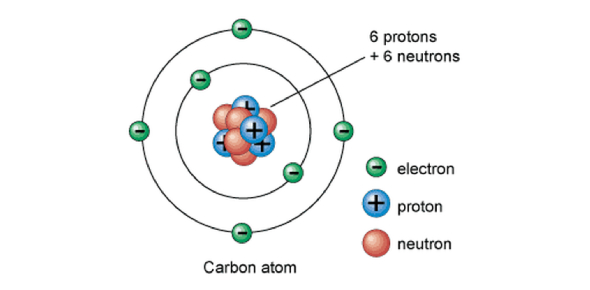# 8th-grade Atomic Structure And The Periodic Table

15 Questions | Total Attempts: 8334SettingsYou’re never too young or too old to start learning about science, and today we’ll be doing so at an eighth-grade level. How much do you know about atomic structure and the periodic table of elements? Take the following quiz and find out for sure!

• 1.
The central part of an atom is called the
• A.

Electron

• B.

Neutron

• C.

Proton

• D.

Nucleus

• 2.
The electric charge on a proton is
• A.

Positive

• B.

Neutral

• C.

Negative

• D.

Changing

• 3.
The number of protons in the nucleus is the
• A.

Atomic mass

• B.

Isotope

• C.

Atomic number

• D.

Half-life

• 4.
Nitrogen has the atomic number 7. An isotope of nitrogen containing seven neutrons would be
• A.

Nitrogen-13

• B.

Nitrogen-14

• C.

Nitrogen-15

• D.

Nitrogen-16

• 5.
How do the size of a negative ion compare to the size of the atom that formed it?
• A.

It's smaller

• B.

It's larger

• C.

It's the same size

• D.

It varies

• 6.
The modern periodic table is organized by
• A.

Size of atom

• B.

Atomic mass

• C.

Number of neutrons

• D.

Atomic number

• 7.
Elements in a group have
• A.

A wide range of chemical properties

• B.

• C.

Similar chemical properties

• D.

The same number of protons

• 8.
Elements in a period have
• A.

A wide range of chemical properties

• B.

• C.

Similar chemical properties

• D.

The same number of protons

• 9.
From left to right in a period, the size of the atom
• A.

Increases

• B.

Decreases

• C.

Remains the same

• D.

Shows no pattern

• 10.
The elements in Group 1 of the periodic table are commonly called the
• A.

Alkali metals

• B.

Transition metals

• C.

Alkaline earth metals

• D.

Rare earth metals

• 11.
The isotope nitrogen-13 has a half-life of 10 minutes. If you start with 40 grams of this isotope, how many grams will you have left after twenty minutes?
• A.

10

• B.

15

• C.

20

• D.

30

• 12.
What does the number above the symbol for each element represent?
• A.

The number of isotopes

• B.

Its atomic number

• C.

Its number of neutrons

• D.

Its atomic mass

• 13.
The atom of what element is in Period 4, Group 13?
• A.

Na

• B.

Ga

• C.

Al

• D.

K

• 14.
What do the elements on the far right of the table (He, Ne, Ar, and Kr) have in common?
• A.

They do not generally react with other elements

• B.

They are liquid in normal conditions

• C.

They are metals that rust easily

• D.

They are very reactive gases

• 15.
How many electrons does a neutral chlorine (Cl) atom contain
• A.

16

• B.

17

• C.

18

• D.

19

Related TopicsBack to top